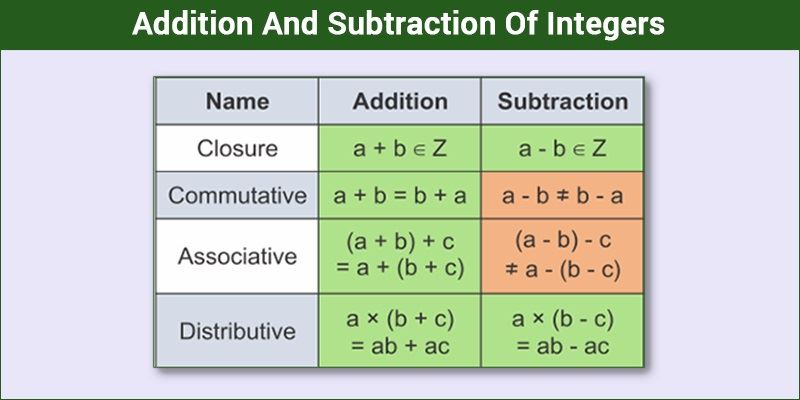# Integer Worksheet

These integers worksheets are an effort from BYJU’S to provide students with a platform where they can test their competence in the topic and get a clear idea of where they stand. These worksheets also help students to prepare for examinations and tests as they would be able to go through the complete topic at one go.

The worksheets have been prepared by trained teachers and are incredibly reliable. Students preparing for CBSE Class 7 examination can use these integers worksheet to attain a bulletproof preparation. This worksheet will have all types of integer-related problems.

Topics such as Addition of Integers, Subtraction of Integers and how to simplify the said integers for class 6 are explored in this maths worksheet. Various types of problems, such as word problems and problems based on activities, are also explored. Setting the integers in order and comparing them is also explored in detail, along with multiple-choice questions based on concepts of opposite value and absolute value.Multiplying and dividing integers is also a section which receives a lot of attention from the paper setters. Practising these sheets using the math formulas will give the students the confidence to tackle all kinds of questions, and they can achieve better results by first going through the chapter, revising the major concepts and then practising these questions.

Be aware of the time you are taking to solve these questions as that is the factor that makes and breaks the exam performance of students. The speed at which they work in the comfort of their home hugely varies from the speed required to attempt the entire questionnaire in the examination so time yourself. These worksheets explore all the different types of problems in all the various topics under the heading of Integers in detail for the benefit of students.

## Integers Worksheet for Class 6

1. Write all integers between a) 0 and 6,b) -3 and 3
2. Using the number line, write the integer which is 6 less than 2.
3. Write 5 negative integers more than -7.
5. Find the sum of -8, 23, -32, -17 and -63.

Integers worksheet PDF for class 6 to solve more problems:-Download PDF Here

## Integers Worksheet for Class 7

1. Divide 324 by -27.
2. Find the value of [32 +2 × 17 + (-6)] ÷ 15
3. Fill in the blanks: ______ ÷ 567 = -1
4. Simplify: (-5)-(-48) ÷ (-16) + (-2) × 3
5. Solve: 22 – ¼ {-5-(-48) ÷ (-16)}

Integers worksheet PDF for class 7 to solve more problems:-Download PDF Here

1. Jaiditya gupta

I want my more practice sheets on the chapter INTEGERS of class 6

• lavanya

• MOKSHITA JAIN

u can visit online site of byjuse to veiw worksheets

2. Aayush Biswas

I want the solutions of the integers worksheet

3. Provide more objective questions or MCQ .Seeing the present online exams.

4. solutions for worksheet of class 7 chapter1

5. Hello I am inaya
I am having my exam of maths on 21st July , 2020 .

So I want to give self assessment test of class 7th maths ch 1 of bodmas formula how to use in integers.

So can u create that.

6. Please give some more questions for practice

7. Rakshitha

Hai i am Rakshitha i std in 7th std please give work sheet of integers